Courses

# Streamflow Measurement - 2

## 10 Questions MCQ Test Topicwise Question Bank for Civil Engineering | Streamflow Measurement - 2

Description
This mock test of Streamflow Measurement - 2 for Civil Engineering (CE) helps you for every Civil Engineering (CE) entrance exam. This contains 10 Multiple Choice Questions for Civil Engineering (CE) Streamflow Measurement - 2 (mcq) to study with solutions a complete question bank. The solved questions answers in this Streamflow Measurement - 2 quiz give you a good mix of easy questions and tough questions. Civil Engineering (CE) students definitely take this Streamflow Measurement - 2 exercise for a better result in the exam. You can find other Streamflow Measurement - 2 extra questions, long questions & short questions for Civil Engineering (CE) on EduRev as well by searching above.
QUESTION: 1

Solution:
QUESTION: 2

### In a river carrying a discharge of 142 m3/s, the stage at a station A was 3,6 m and the water surface slope was 1 in 6000. If during a flood, the stage at A was 3.6 m and the water surface slope was 1 in 3000, what was the flood discharge (approximately)?

Solution:

Discharge is directly proportional to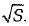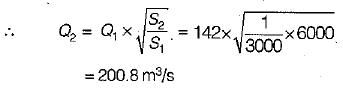QUESTION: 3

### For a given stream the rating curve applicable to a section is available. To determine the discharge in this stream, the following data are needed

Solution:
QUESTION: 4

The stage of river carrying a discharge of Q m3/sec at a point is 10 m and slope of water surface is (1/4000). The discharge of a flood at the same point and same stage of 10 m with a water surface slope of (1/1000) will be

Solution:
QUESTION: 5

When a routine and regular information of the discharge of a stream is desired, it is usually obtained

Solution:
QUESTION: 6

As floodwave passess a given section of a river, life lime of occurrence of the maximum stage and that maximum discharge will be such that

Solution:
QUESTION: 7

The surface velocity at any vertical section of a stream is

Solution:
QUESTION: 8

Calibration of a current meter for use, in channel flow measurement is done in a

Solution:

The relation between the stream velocity and revolutions per second of the current meter is called the calibration equation. The calibration equation is unique to each instrument and is determined by towing the instrument in a special tank called towing tank. Towing' tank is a long channel containing still water, with arrangements for moving a carriage longitudinally over its surface at constant speed. The current meter to be calibrated is mounted on the carriage with the rotating element immersed to a specified depth in the water body in the tank. The carriage is then towed at a predetermined constant speed(v) and the corresponding average value of revolutions per second (Ns) of the instrument determined. This experiment is repeated over the complete range of velocities and a best-fit linear relation in the form of the equation v = aNs + b where a, b are constants of the current meter.

QUESTION: 9

If Vs = surface velocity of a stream at a vertical, the average velocity V in the vertical will be about

Solution:
QUESTION: 10

In the case of large rivers, a number of equidistant vertical sections of the total width of flow are identified, for the purpose of finding by numerical integration, the total discharge on any day. On each section, the mean velocity is taken as the arithmetic average of two typical depths on that section. Then the mean velocity is worked out for that section. Usually, the mean velocity of any section, corresponds to which one of the following?
(V represents the point velocity at the given section and the depth such as 0.1 d, 0.2d.... etc.)

Solution:

In shallow stream of depth up to about 3.0 m, the average velocity,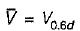This is called single point observation.
In moderately deep streams the velocity is observed at two points.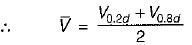In rivers having large flood flows, only surface velocity (Vs) is measured within a depth of about 0.5 m from the surface. The average velocity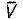= kVs where k is in the range of 0.85 to 0.95.